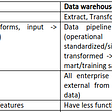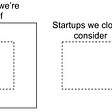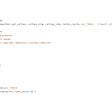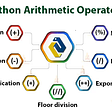# Financial Risk Assessment with Python

In this article I will be stepping through Python code that I wrote to help analyze companies stocks within a pool of their competitors.

# The Finance

## Simple Moving Average:

The strategy being used will not tell you exactly which stock to pick or what price a stock will be at in the future. It is simply a way of comparing companies that are similar.

We will be using a SMA (Simple Moving Average) to determine how volatile a stock is. A Simple moving average can be calculated by taking any number of consecutive closing prices and dividing them by the period you chose.

The period of the SMA can be any natural number really (N > 0), but the most useful SMA values fall between 20–200 depending on the timeframe that is being used. A lower SMA value will respond faster to changes in the underlying price.

It is important to note that because our number of daily closing prices is finite there will be instances where the SMA cannot be calculated (i.e.; there are not enough future underlying prices to calculate the appropriate SMA). We will have to take this into account when writing the code.

## Residual:

In order to turn the SMA values calculated into a data that is interpretable, we will be calculating the difference between the underlying and the SMA value at each point where both are defined.

Then we can sum the differences and divide the sum by the number of values we found. This will give us a raw value that could potentially be compared to those of other similar companies.

# The Code

In the Highest of levels, we need to:

1. Decide who we will be analyzing and when we want to analyze them.
2. Establish a data source to retrieve adjusted closing prices for each day within the chosen period.
3. Plot the Underlying vs. The SMA for visualization.
4. Calculate Average of Residuals as explained previously.

Let's begin by importing the necessary packages:

datetime will allow our program to read our specified time period.

Pandas_datareader.data as web helps us extract data from the Web.

All three variations of Matplotlib will allow us to actually graph and visualize the data that we are interpreting.

Functools will let us reduce our array of residual differences to one total Sum.

`import datetimeimport pandas_datareader.data as webimport matplotlib.pyplot as pltfrom matplotlib import styleimport functoolsimport matplotlib as mpl`

Next, we can initialize and set the time periods we want to analyze. I chose a two year period for a more simple data visualization:

`# Initialize and set the time periods that we want to analyzestart = datetime.datetime(2017, 1, 1)end = datetime.datetime(2019, 11, 2)`

Next, we should pick the companies that we want to compare. I chose Apple, Microsoft, and Netflix. These companies have relatively similar underlying prices at the publication date of this article:

`# make a list of companies that should be tested# Apple: AAPL, Microsoft: MSFT, Netflix: NFLXstock_tickers = ['AAPL', 'MSFT', 'NFLX']`

Now we will enter our main loop that plots the stock charts along with the Simple Moving Averages. I chose a 20 day period for my SMA soely for data visualization:

`# Iterate over each company in the list and produce:# 1. Graph of Moving average vs stock price# 2. Average of the residual for each day moving average can be calculatedfor i in range(len(stock_tickers)):    # Read our data from Yahoo Finance    df = web.DataReader(stock_tickers[i], 'yahoo', start, end)    df.tail()    # Create a list of the closing prices for each day     close_price = df['Adj Close']    # Calculate the SMA for each ticker with a 20 day period    moving_avg = close_price.rolling(window=20).mean()`

Now we must Plot the values that we have calculated (This is still contained within the original ‘for’ loop). I used plt.show() to physically show my graphs. If you are using a Collab Notebook like Jupyter, you can use %matplotlib inline to display graphs.

`# Adjusting the size of matplotlibmpl.rc('figure', figsize=(8, 7))# Adjusting the style of matplotlibstyle.use('ggplot')# Plot the Moving Average vs. Closing Priceclose_price.plot(label=stock_tickers[i])moving_avg.plot(label='moving_avg')plt.legend()plt.show()`

From Matplotlib we receive these graphs. The blue line represents the Simple Moving Average with a 20 day period:

Now to make use of this SMA data, we will calculate the average of the residuals (This is still contained within the original for loop). It is important to note that because the number of days we can collect closing prices for is finite and bounded, some values will result in ‘NaN’ which means Not a Number. These values are eliminated from the parsed list through a mini function called lambda. This is comparable to the Lambda Function notation in Java or Arrow Function in JavaScript.

`# Finally, Sum the the Residuals of Moving Average ~ Closing Pricedifferences = []# Iterate over each list and calculate differencesfor j in range(len(close_price)):    x = abs(moving_avg[j] - close_price[j])    differences.append(x)# Eliminate the values that are of type 'nan'cleanedList = [x for x in differences if str(x) != 'nan']# Use the Reduce method and a short form function 'lambda' combine all elements of the listcombined_value = functools.reduce(lambda a, b: a + b, cleanedList)# Calculate the average of the residualsaverage = combined_value / len(cleanedList)print("The residual average of " + stock_tickers[i] + " is: " + str(average))`

The final output yields:

`The residual average of AAPL is: 5.875529758261265The residual average of MSFT is: 2.0096710682601384The residual average of NFLX is: 12.446982918608967`

# Analysis and Conclusion

The purpose of this program and article is not necessarily to help people trade but rather to show people how they can leverage Python to analyze large amounts of data.

According to my results, the least volatile stock is Microsoft with an average residual value of roughly 2. Does this translate well into the market? … I have no clue haha.

Potential Improvements:

1. My numbers are not Normalized ! There is no scale to the numbers that I produced … therefore I picked stocks with relatively similar underlying prices. You could fix this by finding the standard deviation of Residual Differences.
2. There are more intricate methods for solving this problem already available: Bollinger Bands (John Bollinger) were invented in the 1980’s with this exact concept in mind. You could compare this method with Bollinger Bands or train a model to mirror Bollinger Bands.

--

--

--

## More from Analytics Vidhya

Analytics Vidhya is a community of Analytics and Data Science professionals. We are building the next-gen data science ecosystem https://www.analyticsvidhya.com

## How to Clean Text Data## Book Writing Pattern Analysis## Design Principles and Best Practices: Data engineering (D.E) is not Software engineering (S.E).## Are you sure you’re building predictive models?## Statistical Tests and their Uses## On testing and causal statements## Visualizing Pitcher Clusters: A Next OnAir Digital Experience## Finding startups — notes on work as a new data scientist in an early-stage VC fund## GENERATION OF CYCLONE TRACKS USING WRF MODEL OUTPUT## Why Python for Fintech Companies?## What is Streamlit?## Arithmetic Operators in Python